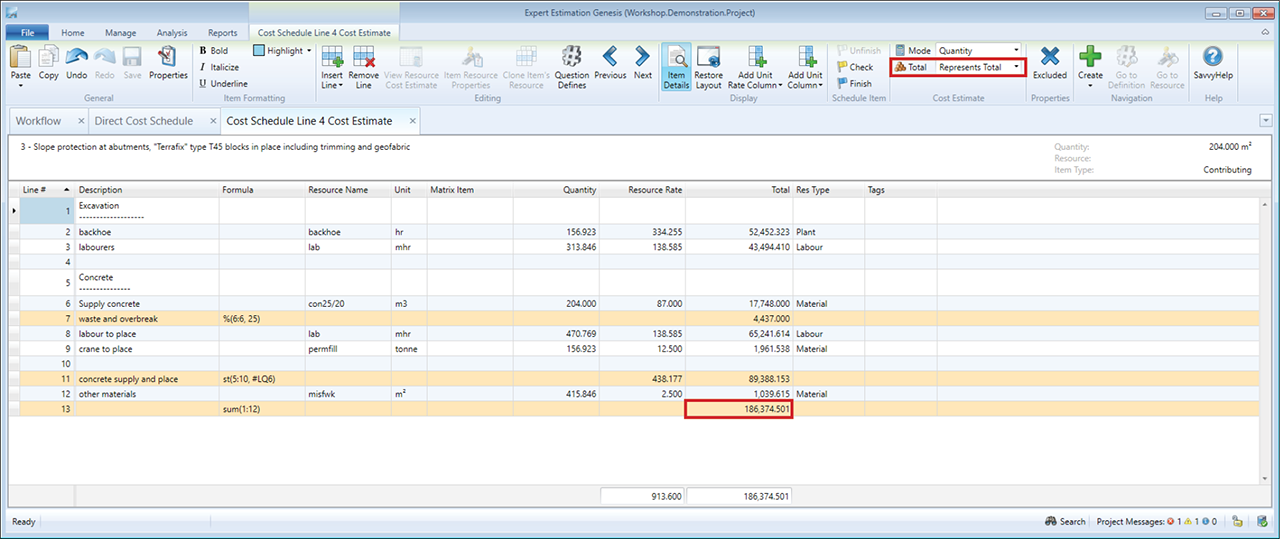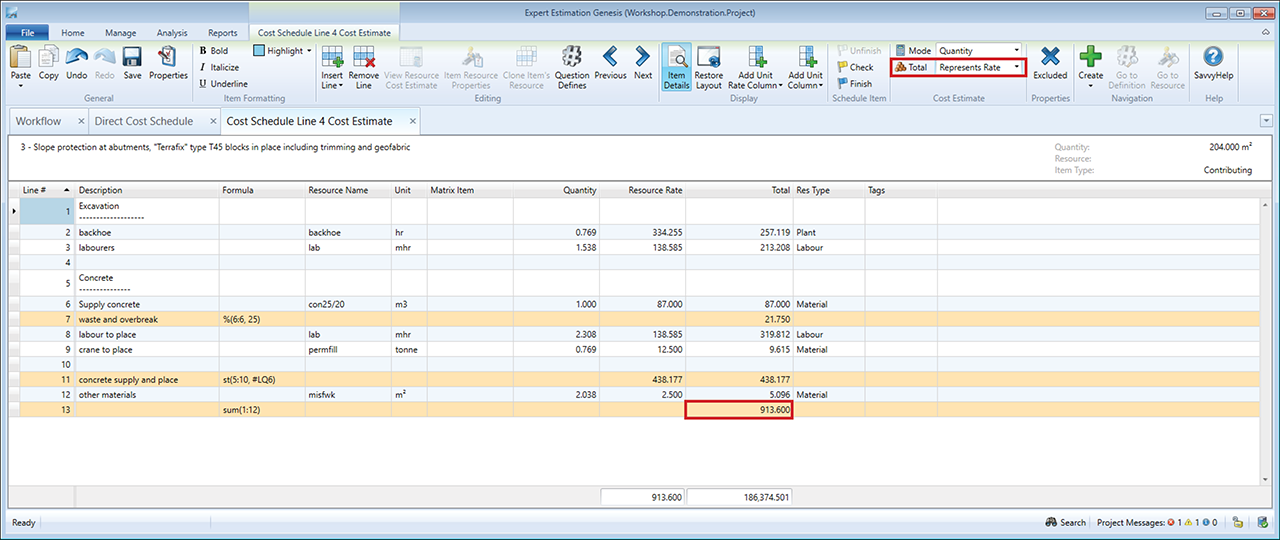## Cost Estimate Calculation Methods

Genesis and Express editions only.

Cost Estimates, for both Direct and Overhead Cost Schedule Items, provide you with the ability to decide what the total of the Cost Estimate will be used for/represented as on the Cost Schedule.

The Total of these lines can be set to represent either the Estimated Rate or Estimated Total for a Cost Schedule item. This change is made by selecting either Represents Total or Represents Rate from the Total drop-down list in the Cost Estimate ribbon menu.

#### REPRESENTS TOTAL

By default, each new cost estimate created uses the Represents Total option. Represents Total is used where the Cost Estimate specifies the quantity of resources required to complete the entirety of a Cost Schedule Item.Cost Estimate window – Represents Total on Cost Schedule

When this option is selected, the associated Schedule Item’s Estimated Total equates to the Cost Estimate’s total. The Estimated Rate is then calculated by dividing the Estimated Total by the Cost Schedule Item’s Quantity.

#### REPRESENTS RATE

The Represents Rate option or Unit Rate estimating mode is where the Cost Estimate specifies the quantity of resources required to complete one unit of a Cost Schedule Item.Cost Estimate window – Represents Rate on Cost Schedule

When this option is selected, the Total of the Cost Estimate equates to the Estimated Rate of the associated Schedule Item. The Estimated Total is then calculated by multiplying the Estimated Rate by the Cost Schedule Item’s Quantity.